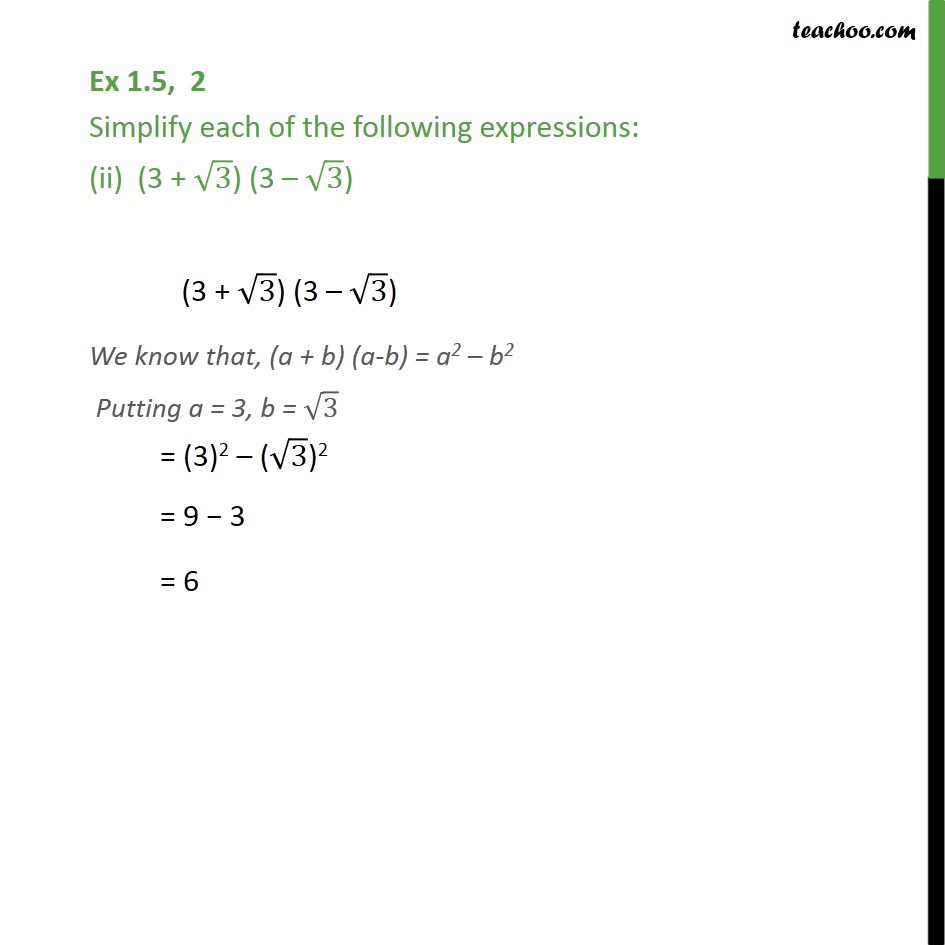Ex 1.4

Chapter 1 Class 9 Number Systems
Serial order wiseLearn in your speed, with individual attention - Teachoo Maths 1-on-1 Class

### Transcript

Ex 1.4, 2 Simplify each of the following expressions: (ii) (3 + 3) (3 3) (3 + 3) (3 3) We know that, (a + b) (a-b) = a2 b2 Putting a = 3, b = 3 = (3)2 ( 3)2 = 9 3 = 6